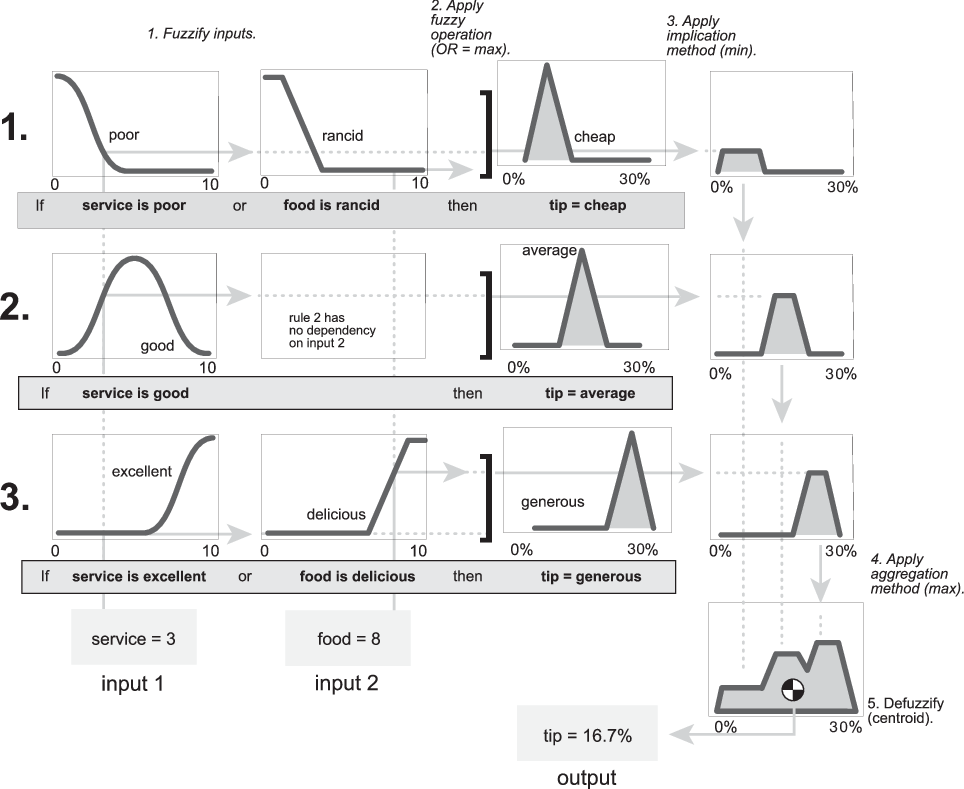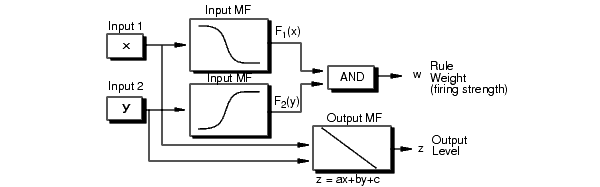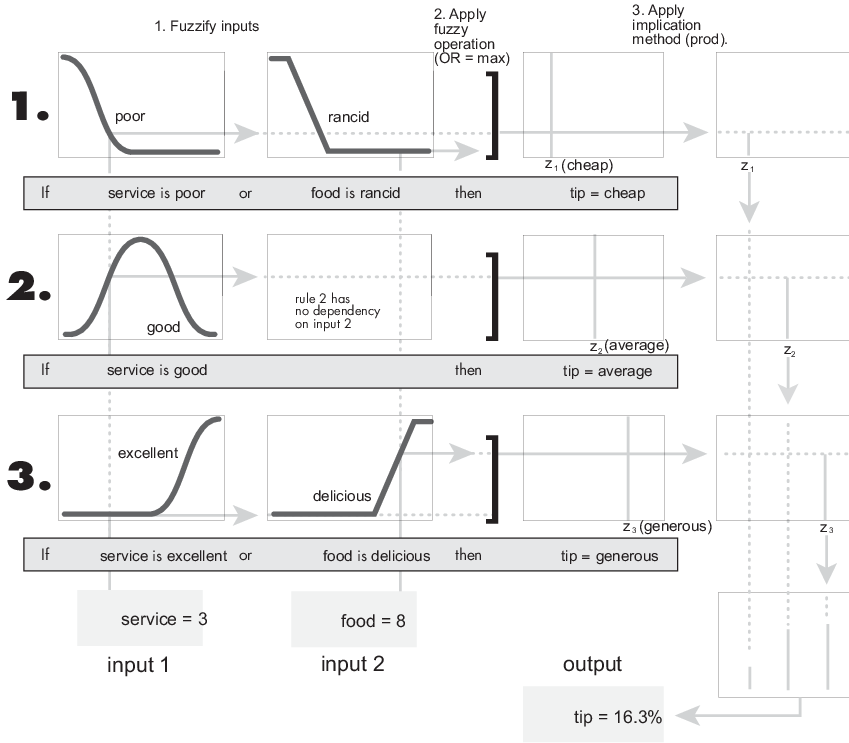## Mamdani and Sugeno Fuzzy Inference Systems

Fuzzy Logic Toolbox™ software supports two types of fuzzy inference systems:

• Mamdani systems

• Sugeno systems

Mamdani
• Intuitive

• Well-suited to human input

• More interpretable rule base

Sugeno
• Computationally efficient

• Work well with linear techniques, such as PID control

• Work well with optimization and adaptive techniques

• Guarantee output surface continuity

• Well-suited to mathematical analysis

### Mamdani Fuzzy Inference Systems

Mamdani fuzzy inference was first introduced as a method to create a control system by synthesizing a set of linguistic control rules obtained from experienced human operators . In a Mamdani system, the output of each rule is a fuzzy set.

Since Mamdani systems have more intuitive and easier to understand rule bases, they are well-suited to expert system applications where the rules are created from human expert knowledge, such as medical diagnostics.

The inference process of a Mamdani system is described in Fuzzy Inference Process and summarized in the following figure.The output of each rule is a fuzzy set derived from the output membership function and the implication method of the FIS. These output fuzzy sets are combined into a single fuzzy set using the aggregation method of the FIS. Then, to compute a final crisp output value, the combined output fuzzy set is defuzzified using one of the methods described in Defuzzification Methods.

### Sugeno Fuzzy Inference Systems

Sugeno fuzzy inference, also referred to as Takagi-Sugeno-Kang fuzzy inference, uses singleton output membership functions that are either constant or a linear function of the input values. The defuzzification process for a Sugeno system is more computationally efficient compared to that of a Mamdani system, since it uses a weighted average or weighted sum of a few data points rather than compute a centroid of a two-dimensional area. 

You can convert a Mamdani system into a Sugeno system using the `convertToSugeno` function. The resulting Sugeno system has constant output membership functions that correspond to the centroids of the Mamdani output membership functions.

Each rule in a Sugeno system operates as shown in the following diagram, which shows a two-input system with input values x and y.Each rule generates two values:

• zi — Rule output level, which is either a constant value or a linear function of the input values:

`${z}_{i}={a}_{i}x+{b}_{i}y+{c}_{i}$`

Here, x and y are the values of input 1 and input 2, respectively, and ai, bi, and ci are constant coefficients. For a zero-order Sugeno system, zi is a constant (a = b = 0).

• wi — Rule firing strength derived from the rule antecedent

`${w}_{i}=AndMethod\left({F}_{1}\left(x\right),{F}_{2}\left(y\right)\right)$`

Here, F1(...) and F2(...) are the membership functions for inputs 1 and 2, respectively.

The output of each rule is the weighted output level, which is the product of wi and zi.

The easiest way to visualize first-order Sugeno systems (a and b are nonzero) is to think of each rule as defining the location of a moving singleton. That is, the singleton output spikes can move around in a linear fashion within the output space, depending on the input values. The rule firing strength then defines the size of the singleton spike.

The final output of the system is the weighted average over all rule outputs:

where N is the number of rules.

The following figure shows the fuzzy inference process for a Sugeno system.Note

Sugeno systems always use product implication and sum aggregation.

Because of the linear dependence of each rule on the input variables, the Sugeno method is ideal for acting as an interpolating supervisor of multiple linear controllers that are to be applied, respectively, to different operating conditions of a dynamic nonlinear system. For example, the performance of an aircraft may change dramatically with altitude and Mach number. Linear controllers, though easy to compute and suited to any given flight condition, must be updated regularly and smoothly to keep up with the changing state of the flight vehicle. A Sugeno fuzzy inference system is suited to the task of smoothly interpolating the linear gains that would be applied across the input space; it is a natural and efficient gain scheduler. Similarly, a Sugeno system is suited for modeling nonlinear systems by interpolating between multiple linear models.

 Mamdani, E.H., and S. Assilian. ‘An Experiment in Linguistic Synthesis with a Fuzzy Logic Controller’. International Journal of Man-Machine Studies 7, no. 1 (January 1975): 1–13. https://doi.org/10.1016/S0020-7373(75)80002-2.

 Sugeno, Michio, ed. Industrial Applications of Fuzzy Control. Amsterdam ; New York : New York, N.Y., U.S.A: North-Holland ; Sole distributors for the U.S.A. and Canada, Elsevier Science Pub. Co, 1985.